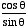index: click on a letter A B C D E F G H I J K L M N O P Q R S T U V W X Y Z A to Z index index: subject areas numbers & symbols sets, logic, proofs geometry algebra trigonometry advanced algebra & pre-calculus calculus advanced topics probability & statistics real world applications multimedia entrieswww.mathwords.com about mathwords website feedback

 cotangent cot ctg The trig function cotangent, written cot θ. cot θ equalsor. For acute angles, cot θ can be found by the SOHCAHTOA definition, shown below on the left. The circle definition, a generalization of SOHCAHTOA, is shown below on the right. f(x) = cot x is a periodic function with period π.See also

 this page updated 19-jul-17 Mathwords: Terms and Formulas from Algebra I to Calculus written, illustrated, and webmastered by Bruce Simmons Copyright © 2000 by Bruce Simmons All rights reserved